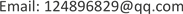1重庆华渝电气集团有限公司，重庆

2西北机电工程研究所，陕西 西安1. 引言

2. 雪崩三极管脉冲发生器

i = i R + i A (1)

U C E = V C C - i R R C (2)

(3)

U C E = u c ( 0 ) − 1 / C ∫ 0 t ( [ i + ( U C E − V C C ) / R L ] d t − [ i + ( U C E − V C C ) / R L ] R L ) (4)

U C E = E C C − i R L (5)

E C = u c ( 0 ) - ∫ 0 t i d t (6)

3. 微波三极管脉冲发生器

U ( ∝ ) = 0 V , U ( 0 ) = 5 V , V ( t ) = 0.5 U ( 0 ) = 2.5 V

4. 数字逻辑器件脉冲发生器

5. 基于DCM的数字电路脉冲发生器

6. 分析与比较

7. 总结